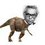# Synthetic Geometry Group-Nihar's Proposal

These problems are my submissions to Xuming's Geometry group.These problems are taken from previous RMO papers so that some of my friends who are preparing for RMO will be benefited by this discussion and thereby prepare and improve themselves :)

Q1) Let $AL$ and $BK$ be the angle bisectors in a non-isosceles triangle $ABC,$ where $L$ lies on $BC$ and $K$ lies on $AC.$ The perpendicular bisector of $BK$ intersects the line $AL$ at $M$. Point $N$ lies on the line $BK$ such that $LN$ is parallel to $MK.$ Prove that $LN=NA.$

Q2) Let $ABC$ be a triangle and let $BB_1,CC_1$ be respectively the bisectors of $\angle{B},\angle{C}$ with $B_1$ on $AC$ and $C_1$ on $AB$, Let $E,F$ be the feet of perpendiculars drawn from $A$ onto $BB_1,CC_1$ respectively. Suppose $D$ is the point at which the incircle of $ABC$ touches $AB$. Prove that $AD=EF$Note by Nihar Mahajan
4 years, 12 months ago

This discussion board is a place to discuss our Daily Challenges and the math and science related to those challenges. Explanations are more than just a solution — they should explain the steps and thinking strategies that you used to obtain the solution. Comments should further the discussion of math and science.

When posting on Brilliant:

• Use the emojis to react to an explanation, whether you're congratulating a job well done , or just really confused .
• Ask specific questions about the challenge or the steps in somebody's explanation. Well-posed questions can add a lot to the discussion, but posting "I don't understand!" doesn't help anyone.
• Try to contribute something new to the discussion, whether it is an extension, generalization or other idea related to the challenge.

MarkdownAppears as
*italics* or _italics_ italics
**bold** or __bold__ bold
- bulleted- list
• bulleted
• list
1. numbered2. list
1. numbered
2. list
Note: you must add a full line of space before and after lists for them to show up correctly
paragraph 1paragraph 2

paragraph 1

paragraph 2

[example link](https://brilliant.org)example link
> This is a quote
This is a quote
    # I indented these lines
# 4 spaces, and now they show
# up as a code block.

print "hello world"
# I indented these lines
# 4 spaces, and now they show
# up as a code block.

print "hello world"
MathAppears as
Remember to wrap math in $$ ... $$ or $ ... $ to ensure proper formatting.
2 \times 3 $2 \times 3$
2^{34} $2^{34}$
a_{i-1} $a_{i-1}$
\frac{2}{3} $\frac{2}{3}$
\sqrt{2} $\sqrt{2}$
\sum_{i=1}^3 $\sum_{i=1}^3$
\sin \theta $\sin \theta$
\boxed{123} $\boxed{123}$

Sort by:

modern algebra text

- 4 years, 10 months agoimg

Since we know that in a triangle the perpendicular bisector of a side and angular bisector of the angle opposite to this side meet at a point which is concyclic with the vertices of the triangle. Here, angular bisector of $\angle BAK$ and perpendicular bisector of $BK$ meet at $M$. So, $A$, $B$, $M$ and $K$ are concyclic. So, $\angle AMK = \angle ABK$.

But, $LN || MK$. So, $\angle ALN = \angle AMK = \angle ABK = \angle ABN$. So, $A$, $B$, $L$ and $N$ are cyclic. It implies that $\angle NAL = \angle NBL = \angle NBA = \angle ALN$. So, $\angle ALN = \angle ANL$, which implies that $NA = NL$.

- 4 years, 12 months ago

what rank did you get in jee 2016 ?

Yeah , this is the standard solution. At first the problem looks tough. But as you decipher the configuration , you crack it like a left hand's play (provided that you are right handed :P)

- 4 years, 11 months ago

Here's mine @Nihar Mahajan - Surya Prakash's Proposals

- 4 years, 12 months ago

@Surya Prakash @Mehul Arora @Agnishom Chattopadhyay @Alan Yan @Shivam Jadhav @Ambuj Shrivastava @Swapnil Das @Sharky Kesa @Saarthak Marathe @Kushagra Sahni @naitik sanghavi If you are interested in this , please make your proposals soon and await the geometry challenges,discussion!

@Calvin Lin Sorry , for mass tagging. But I think this will encourage the participation :)

- 4 years, 12 months ago

- 4 years, 11 months ago

- 4 years, 12 months ago

:3 :3 :3 :3 :3 :3 :3 :3 :3

- 4 years, 12 months ago

@Calvin Lin @Xuming Liang Here's my submission! I hope that this marvelous group forms and accelerates soon :)

- 4 years, 12 months ago